# Einstein’s equation

E=mc². Everybody knows this formula, but not everyone knows what it is, what it means. How can three letters and an exponent mean so much?

Let’s start by defining those letters:

E is the energy;

m is the mass;

c is a constant, i.e. the speed of light in a vacuum, which corresponds to 299792458 m/s, or approximately 3 · 10^8 m/s;

This means that E is the energy of an object of mass m traveling at the speed of light c. This is the definition of kinetic energy:

The kinetic energy of an object is the energy that it possesses due to its motion.

Source: Wikipedia

But where does this formula come from? Since the object travels at the speed of light, we are talking of uniform linear motion, expressed by the equation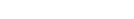where:

s is the space;

v is the velocity;

t-t0 is the time the object moves for (t is the current time and t0 the initial time; example: an objects that starts moving after 3 seconds and stops at t = 8 s;);

s0 is the initial space (for example an object that starts moving at 5 m away from the point of reference);

This is the graph space-time:

As you can see it is a straight line, indicating it is uniform motion, i.e. the velocity is constant.

In our case, since we are talking about speed of light, the equation becomes: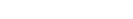Assuming light doesnt’ have an initial time and an initial space, we can write it as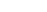We know that energy is measured in J (joules), or, equivalently N · m (Newtons per second), therefore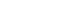where F is the force and s the space.

We also know that F=ma and that a=c/t, hence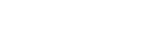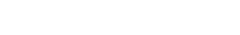s/t is equal to v, c in this case: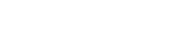Obviously, c · c is simply c², therefore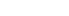This is how we find the famous equation.

This wonderful formula allows us to show that light has energy; light is made of photons which have a mass and, of course, travel at the speed of light. If something has got a mass and moves at a certain speed, it must also have an energy.

The mass of a photon is 3.68 · 10^-51 kg, hence an energy of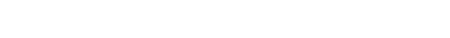As you can see, it has an incredibly small energy, this is why we can’t move object with light. The only ways to increase E would be to increase m and/or c, but they are constant, so it’s not possible. We can introduce another equation for the energy of a photon though, known as the Planck’s equation: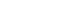where E is still the energy, h is Planck’s constant, approximately equal to 6.626 · 10^-34 m² · kg/s, and v is the Greek letter ni, which is the frequency.

Since both equations express E, we can compare them: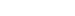This shows that the energy also depends on the frequency, so if we could create a laser with an extremely high frequency we could move objects. This is just my theory though!

Anyway, I really hope that you found this post interesting and if you liked it give it a like, I would really appreciate it!

Join 31 other subscribers.